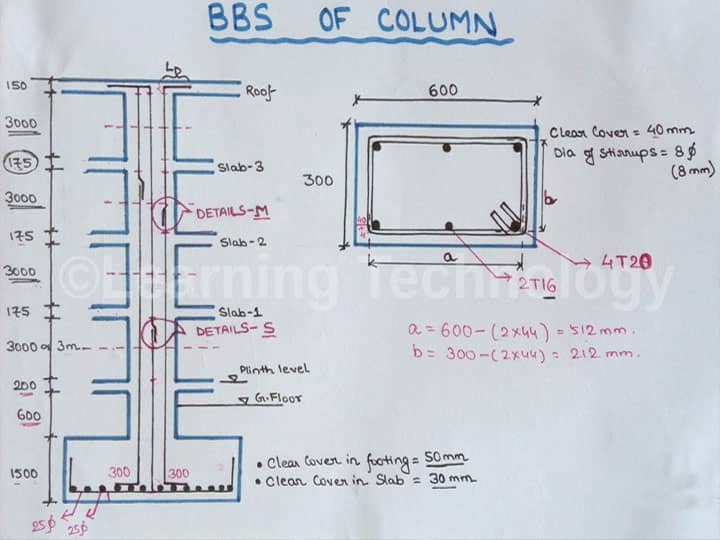Home Articles Calculating the Bar Bending Schedule (BBS) of Columns

# Calculating the Bar Bending Schedule (BBS) of Columns

Welcome back to another BBS discussion. Today, we will examine the bar bending schedule (BBS) for standard columns. A column or pillar in architecture and structural engineering is a structural element that transmits, through compression, the weight of the structure above to other structural elements below. In other words, a column is a compression member.

The term column applies especially to a large round support - the shaft of the column - with a capital and a base or pedestal, which is made of stone, or appearing to be so. A small wooden or metal support is typically called a post, and supports with a rectangular or other non-round section are usually called piers.

The word BBS Plays a significant role in any construction of high-rise buildings. BBS refers to Bar Bending Schedule. Bar Bending Schedule is termed as the calculation of the total steel required for the construction of a building. We use steel to make concrete to be reinforced and for tension requirements. Bar is any type of rebar which is used as a reinforcement in RCC. The bar may be a Mild Steel bar or HYSD bar or TMT Bar.

In Bar bending schedule, the bars are sorted out for each auxiliary unit - beams or columns or sections or footings and so forth - and itemized list is readied which determines the bar area - in footings/pieces/ pillars/columns, bar marking - to recognize the bar as per the drawing, Bar Size - the length of the bar utilized, Quantity - the number of Bars utilized, cutting length, type of Bend and Shape of the bar in fortification drawings.

If you look at a diagram of forces acting on a column, you will see that the column experiences most of the internal forces and moments at the top and bottom, and so much at the center. So, it would be foolish to tie the column at the top or bottom ? always do it at the center.

Best ideas for placing the ties in a column:

1. Always tie the column at the middle.
2. Lap the bars alternately each time, every time.
3. Try to do as little cutting and lapping as possible.
4. Never leave the top and bottom bars alone- always overlap them.
5. There should always be a good amount of spacing between laps.

Now, let us proceed to estimating the BBS of a given column.

## Data given

• Slab Thickness - 125 mm & 100 mm
• Floor height - 3000 mm or 3 m
• Ground Floor Level - 3300 mm
• Footing Height - 300 mm
• Development Length - 50d
• Column has 6 numbers of 20 mmdiabars
• 8mm stirrups @ 150 mm C/C
• Footing Clear Cover - 40 mm
• Slab Clear Cover - 25 mm

## Steps to find the BBS

Utilizing the above data, now we can figure out the BBS of this column.

A. Length of vertical bar
Length of Vertical bar = Development length + Height of Ground Level + Floor Heights + Slab Thicknesses + Overlap Length
= (50?20) +3300+ (3?3000) +(3?125) +100 +(50?20)
= 14775 mm
=14.78 meters

B. Lapping lengths
The lapping length required is 50d
= 50 x Diameter of the bar
= 50 ? 20
=1000 mm

We know that each bar is 12.25 m or 40 feet length (approximately).
Total Length of Vertical Bar
= 14.2 m

This is more than 12.25 m, so, each rod will be lapped at least once to attain the required length.
Therefore, we have added the lapping length with the total length
= 14775+1000
= 15775 mm
=15.78 meters

C. Stirrup cutting length
Length of One Hook = 9d (As per Estimating and Costing in Civil Engineering P.No.214)
Cutting length of Stirrup = Perimeter of stirrup + Number of Bends + Number of Hooks
= 2(a+b) + 3 numbers of90-degreebends + 2 numbers of hooks
= 2(500+200) +(3 x2d) +(2x9d)
=2?700+3x2x20+2x9x20
=1880 mm

D. Numbers of stirrups
The number of stirrups required = (Total length of Column/spacing of stirrups) +1
= (3300+125+3000+125+3000+125+3000+100)/150 + 1
=85 pieces
BBSscheduleof the given column
Vertical bar: 20mmdia, 6 pieces each of 14.2 meters, totaling 85.2 meters
Stirrups bar: 85 pieces of 8mm dia each of 1.88 meter, totaling 159.8 meters